# RD Sharma Solutions Class 9 Chapter 10 Congruent Triangles

Read RD Sharma Solutions Class 9 Chapter 10 Congruent Triangles below, students should study RD Sharma class 9 Mathematics available on Studiestoday.com with solved questions and answers. These chapter wise answers for class 9 Mathematics have been prepared by teacher of Grade 9. These RD Sharma class 9 Solutions have been designed as per the latest NCERT syllabus for class 9 and if practiced thoroughly can help you to score good marks in standard 9 Mathematics class tests and examinations

Exercise 10.1

Question 1: In figure, the sides BA and CA have been produced such that BA = AD and CA = AE. Prove that segment DE ∥ BC.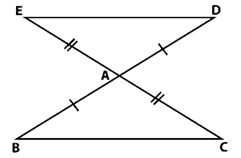Solution:
It is given that,
CA = AE (Given)
∠BAC = ∠DAE (Vertically opposite angle)
∆ABC ≅ ∆ADE (By SAS congruent criterion)
BC = DE (CPCT)
∠C = ∠E and ∠B = ∠D (alternate interior angle)
BC || ED
Hence Prove.

Question 2: In a PQR, if PQ = QR and L, M and N are the mid-points of the sides PQ, QR and RP respectively. Prove that LN = MN.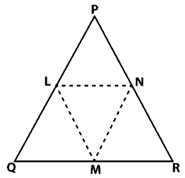Solution:
Draw a figure based on given instruction,
In △PQR,
It is given that,
PQ = QR and L, M, N are midpoints of the sides PQ, QP and RP.
∠QPR = ∠QRP (two sides of the triangle are equal, so △PQR is an isosceles triangle)
L is the midpoint of PQ and M is midpoints QR.
PL = LQ = QM = MR
Now, consider Δ LPN and Δ MRN,
LP = MR (Proved above)
∠LPN = ∠MRN (∠QPR = ∠LPN and ∠QRP = ∠MRN)
PN = NR (N is midpoint of PR)
Δ LPN ≅ Δ MRN (By SAS congruence criterion)
LN = MN (CPCT)
Hence Proved.

Question 3: In figure, PQRS is a square and SRT is an equilateral triangle. Prove that
(i) PT = QT (ii) ∠ TQR = 15°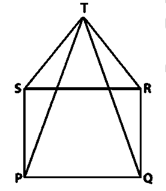Solution:
(i) It is given that, PQRS is a square and SRT is an equilateral triangle.
PQRS is a square
PQ = QR = RS = SP_________(i)
∠SPQ = ∠PQR = ∠QRS = ∠RSP = 90° (Property of a square)
△SRT is an equilateral triangle
SR = RT = TS_________(ii)
∠ TSR = ∠ SRT = ∠ RTS = 60° (Property of equilateral triangle)
From (i) and (ii) we get,
PQ = QR = SP = SR = RT = TS_________(iii)
From figure we get,
∠TSP = ∠TSR + ∠ RSP
∠TSP = 60° + 90°
∠TSP = 150°
∠TRQ = ∠TRS + ∠ SRQ
∠TRQ = 60° + 90°
∠TRQ = 150°
∠TSP = ∠TRQ = 150°__________(iv)
From ΔTSP and ΔTRQ we get,
TS = TR (Proved above)
SP = RQ (Proved above)
∠TSP = ∠TRQ (each 150°)
ΔTSP ≅ ΔTRQ (SAS congruence criterion)
PT = QT (Corresponding parts of congruent triangles)
Hence proved

(ii) In Δ TQR.
QR = TR
ΔTQR is an isosceles triangle.
∠QTR = ∠TQR (angles opposite to equal sides)
∠QTR + ∠ TQR + ∠TRQ = 180° (Sum of angles in a triangle is 180°)
∠TQR + ∠ TQR + ∠TRQ = 180°
2∠TQR + 150° = 180°
2∠TQR = 30°
∠TQR = 15°
Hence proved

Question 4: Prove that the medians of an equilateral triangle are equal.
Solution: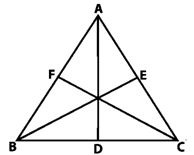It is given that, △ABC is an equilateral and Let D, E, F are midpoints of BC, CA and AB.
Here, AD, BE and CF are medians of △ABC.
D is midpoint of BC
BD = DC
F is midpoint of AB
CE = EA
E is midpoint of AC
AF = FB
ΔABC is an equilateral triangle
AB = BC = CA (equilateral triangle)
BD = DC = CE = EA = AF = FB
∠ABC = ∠BCA = ∠CAB = 60° (angles in a equilateral triangle are 60°)
In ΔABD and ΔBCE
AB = BC (sides of a equilateral triangle)
BD = CE (sides of a equilateral triangle)
∠ABD = ∠BCE (each 60°)
ΔABD ≅ ΔBCE (By SAS congruence criterion)
AD = BE (Corresponding parts of congruent triangles) __________(1)
In ΔBCE and ΔCAF,
BC = CA (sides of a equilateral triangle)
∠BCE = ∠CAF (each 60°)
CE = AF (Proved above)
ΔBCE ≅ ΔCAF (By SAS congruence criterion)
BE = CF (CPCT) ____________(2)
From equation (1) and (2), we get
AD = BE = CF (Median of equilateral triangle)
The medians of an equilateral triangle are equal.
Hence proved

Question 5: In a Δ ABC, if ∠A = 120° and AB = AC. Find ∠B and ∠C.
Solution: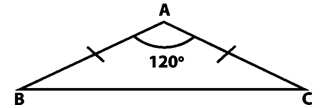ΔABC is an isosceles triangle since AB = AC
∠ B = ∠ C (Angles opposite to equal sides are equal)
∠ A + ∠ B + ∠ C = 180° (Sum of angles in a triangle is 180°)
∠ A + ∠ B + ∠ B = 180°
120° + 2∠B = 180°
2∠B = 180° – 120°
2∠B = 60°
∠ B = 30°
Hence, the value of ∠B = ∠C = 30°.

Question 6: In a Δ ABC, if AB = AC and ∠ B = 70°, find ∠ A.
Solution:
It is given that, in ΔABC, AB = AC and ∠B = 70°
∠ B = ∠ C (Angles opposite to equal sides are equal)
∠ B = ∠ C = 70°
∠ A + ∠ B + ∠ C = 180° (Sum of angles in a triangle is 180°)
∠ A + 70° + 70° = 180°
∠ A = 180° – 140°
∠ A = 40°
Hence, the value of ∠A is 40°.

Question 7: The vertical angle of an isosceles triangle is 100°. Find its base angles.
Solution:
It is given that ΔABC is an isosceles triangle.
AB = AC
Given that vertical angle A is 100°
∠B = ∠C (Angles opposite to equal sides are equal)
∠A + ∠B + ∠C = 180° (Sum of interior angles of a triangle is 180°)
100° + ∠B + ∠B = 180°
2∠B = 180° - 100°
∠B = (80°)/2
∠B = 40°
Hence, the value of base angles ∠B and ∠C is 40°.

Question 8:  In a figure AB = AC and ∠ACD = 105°. Find ∠BAC.
Solution:It is given that,
AB = AC and ∠ACD = 105°
BCD is a Straight line.
∠BCA + ∠ACD = 180°
∠BCA + 105° = 180°
∠BCA = 180° - 105°
∠BCA = 75°
ΔABC is an isosceles triangle.
AB = AC
∠ABC = ∠ ACB (Angles opposite to equal sides are equal)
∠ACB = 75°
∠ABC = ∠ACB = 75°
∠ABC + ∠BCA + ∠CAB = 180° (Sum of Interior angles of a triangle is 180°)
75° + 75° + ∠CAB =180°
150° + ∠BAC = 180°
∠BAC = 180° - 150°
∠BAC = 30°
Hence, the value of ∠BAC is 30°.

Question: 9  Find the measure of each exterior angle of an equilateral triangle.

Solution:

It is given that, ∆ABC equilateral triangle.
AB = BC = CA
∠ABC = ∠BCA = CAB
∠ABC = ∠BCA = CAB = 60° (all angles in a equilateral triangle are equal)
Extend side BC to D,
CA to E
AB to F
BCD is a straight line.
∠BCA + ∠ACD = 180° (Angles made in on it are 180°)
60° + ∠ACD = 180°
∠ACD = 120°
The exterior angle of an equilateral triangle is 120°
Hence proved

Question: 10  If the base of an isosceles triangle is produced on both sides, prove that the exterior angles so formed are equal to each other.
Solution:AB = AC (Sides of a isosceles triangle)
∠B = ∠C (Angles opposite to equal sides are equal)
Exterior angles ∠ACD
∠A + ∠B = ∠ACD _______(1) (Sum of opposite interiors angles)
Exterior angles ∠ACD
∠A + ∠C = ∠ABE _______(2) (Sum of opposite interiors angles)
By equation (1) and (2)
∠A + ∠B = ∠A + ∠C
∠ACD = ∠ABE
Hence proved

Question 11:  In Figure AB = AC and DB = DC, find the ratio ∠ABD : ∠ACD.Solution: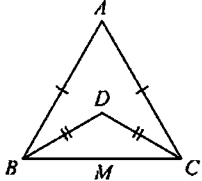In ∆ABC
AB = AC
∠ABC = ∠ACB (Angles opposite to equal sides are equal)
In ∆DBC
DB = DC
∠DBC = ∠DCB (Angles opposite to equal sides are equal)
∠ABC = ∠ABD + ∠DBC
∠ABC - ∠DBC = ∠ABD
∠ACB = ∠ACD = ∠DCB
∠ACB - ∠DCB = ∠ACD
(∠ABC)/(∠ACD)=(∠ABC-∠DBC)/(∠ACB-∠DCB)
(∠ABC)/(∠ACD)=(∠ABC-∠DBC)/(∠ABC-∠DBC)
(∠ABC)/(∠ACD)=1/1
∠ABC∶ ∠ACD=1:1

Question: 12  Determine the measure of each of the equal angles of a right-angled isosceles triangle.
OR
ABC is a right-angled triangle in which ∠A = 90° and AB = AC. Find ∠B and ∠C.
Solution:
ABC is a right angled triangle.
Consider on a right - angled isosceles triangle ABC such that
∠A = 90°
AB = AC
∠C = ∠B _______(i) (Angles opposite to equal sides are equal)
∠A + ∠B + ∠C =180° (Sum of angles in a triangle is 180°)
90° + ∠ B+ ∠ B = 180°
2∠B = 90°
∠B = 45°
∠B = 45°
∠C = 45°
Hence, the angles of a right-angled isosceles triangle is 45°

Question: 13  AB is a line segment. P and Q are points on opposite sides of AB such that each of them is equidistant from the points A and B (See Fig. (10.26). Show that the line PQ is perpendicular bisector of AB.Solution: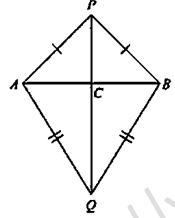It is given that,
AB is line segment and P, Q are points.
AP = BP _________(i)
AQ = BQ ________(ii)
We have to prove that PQ is perpendicular bisector of AB.
In ΔPAQ and ΔPBQ,
AP = BP (Proved above)
AQ = BQ (Proved above)
PQ - PQ (Common)
Δ PAQ ≅ Δ PBQ (by SSS congruence) ________(iii)
∠APC = ∠BPC (CPCT)
Now, we can observe that ∆APB and ∆ABQ are isosceles triangles. [From (i) and (ii)]
∠PAB = ∠ABQ (Angles opposite to equal sides are equal)
∠QAB = ∠QBA (Angles opposite to equal sides are equal)
In ΔPAC and ΔPBC
AP = BP (Proved above)
∠APC = ∠BPC (Proved above by equation 3)
PC = PC (common side)
ΔPAC ≅ ΔPBC (SAS congruency of triangle)
AC = CB (CPCT)
∠PCA = ∠PBC (CPCT)
ACB is Straight line
∠ACP + ∠ BCP = 180°
∠ACP = ∠PCB
∠ACP = ∠PCB = 90°
AC = CB, C is the midpoint of AB
PC is the perpendicular bisector of AB
Hence, C is a point on the line PQ, PQ is the perpendicular bisector of AB.

Exercise 10.2

Question 1: In figure, it is given that RT = TS, ∠ 1 = 2∠2 and ∠4 = 2∠3. Prove that ΔRBT ≅ ΔSAT.Solution:
It is given that,
RT = TS
∠1 = 2 ∠2
∠4 = 2∠3
Need to prove: ΔRBT ≅ ΔSAT
RB and SA intersect each other at point O.
∠AOR = ∠BOS (Vertically opposite angles)
∠1 = ∠4
2∠2 = 2∠3 (Given)
∠2 = ∠3
In ΔTRS,
RT = TS
ΔTRS is an isosceles triangle
∠TRS = ∠TSR (Angles opposite to equal sides are equal)
∠TRS = ∠TRB + ∠2_______(1)
∠ TSR = ∠TSA + ∠3_______(2)
From equation (1) and (2) we get
∠TRB + ∠2 = ∠TSA + ∠3
∠TRB = ∠TSA (∠2 = ∠3)
In ΔRBT and ΔSAT
RT = ST (Given)
∠TRB = ∠TSA (Proved above)
∠T = ∠T (common)
Δ RBT ≅ Δ SAT (ASA criterion of congruence)
Hence Prove.

Question 2: Two lines AB and CD intersect at O such that BC is equal and parallel to AD. Prove that the lines AB and CD bisect at O.
Solution: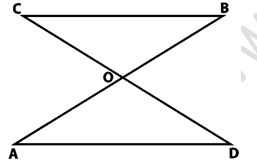Need to prove: AB and CD bisect at O.
In ΔAOD and ΔBOC
∠OCB =∠ODA (alternate interior angles)
∠OBC = ∠OAD (alternate interior angles)
Δ AOD ≅ Δ BOC (ASA Criterion)
OA = OB (By CPCT)
OD = OC (By CPCT)
AB and CD bisect each other at O.
Hence Proved.

Question 3: BD and CE are bisectors of ∠B and ∠C of an isosceles Δ ABC with AB = AC. Prove that BD = CE.
Solution:It is given that,
AB = AC
∠ABC = ∠ACB________(i) (Angles opposite to equal sides are equal)
BD is bisector of ∠B
∠ABD = ∠DBC
CE is bisectors of ∠C
∠BCE = ∠ECA
In ΔEBC and ΔDCB we get,
∠EBC = ∠DCB (Proved above)
BC = BC (Common)
∠BCE = ∠CBD (Proved above)
ΔEBC ≅ ΔDCB (By ASA congruence criterion)
CE = BD (CPCT)
BD = CE (CPCT)
Hence proved

Exercise 10.3

Question 1: In two right triangles one side an acute angle of one are equal to the corresponding side and angle of the other. Prove that the triangles are congruent.
Solution:It is given that, two right triangles one side an acute angle of one are equal to the corresponding side and angle of the other.
In right triangle △ABC and △DEF, we get
∠B = ∠E (each 90°)
AB = DE (give)
∠C = ∠F (give)
ΔABC ≅ ΔDEF (By AAS congruence criterion)
Both the triangles are congruent.
Hence proved

Question 2: If the bisector of the exterior vertical angle of a triangle be parallel to the base. Show that the triangle is isosceles.
Solution:
Let ABC be a triangle such that AD is the angular bisector of exterior vertical angle, ∠EAC and AD ∥ BC.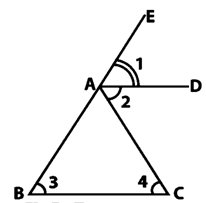From figure,
∠1 = ∠2 (as AD is a bisector of ∠EAC) _______(i)
∠2 = ∠4 (alternative angle) _______(ii)
∠1 = ∠3 (corresponding angles) _______(iii)
From equation (i), (ii) and (iii), we get
∠3 = ∠4
AB = AC
ΔABC is an isosceles triangle.

Question 3: In an isosceles triangle, if the vertex angle is twice the sum of the base angles, calculate the angles of the triangle.
Solution:
It is given that, ΔABC be isosceles.
AB = AC
∠B = ∠C
Vertex angle A is twice the sum of the base angles B and C.
∠A = 2(∠B + ∠C)
∠A = 2(∠B + ∠B)
∠A = 2(2∠B)
∠A = 4∠B
∠A + ∠B + ∠C = 180° (sum of angles in a triangle is 180°)
4∠B + ∠B + ∠B = 180°
6∠B =180°
∠B = 30°
∠B = ∠C
∠B = ∠C = 30°
∠A = 4∠B
∠A = 4 x 30°
∠A = 120°
Hence, the value of angles of the given triangles are 30° and 30° and 120°.

Question 4: PQR is a triangle in which PQ = PR and is any point on the side PQ. Through S, a line is drawn parallel to QR and intersecting PR at T. Prove that PS = PT.
Solution:
It is given that, PQR is a triangle such that PQ = PR and S is any point on the side PQ and ST ∥ QR.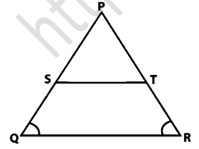Here,
PQ = PR
So, △PQR is an isosceles triangle.
∠PQR = ∠PRQ
∠PST = ∠PQR (Corresponding angles)
∠PTS = ∠PRQ (Corresponding angles)
As, ∠PQR = ∠PRQ
∠PST = ∠PTS
In Δ PST,
∠ PST = ∠ PTS
Δ PST is an isosceles triangle.
Hence, PS = PT.
Hence proved

Exercise 10.4
Question 1: In figure, It is given that AB = CD and AD = BC. Prove that ΔADC ≅ ΔCBA.Solution:
It is given that,
AB = CD and AD = BC
AB = CD (Given)
And AC = AC (Common)
ΔADC ≅ ΔCBA (by SSS congruence criterion)
Hence proved

Question 2: In a Δ PQR, if PQ = QR and L, M and N are the mid-points of the sides PQ, QR and RP respectively. Prove that LN = MN.
Solution:
It is given that, in ΔPQR, PQ = QR and L, M and N are the mid-points of the sides PQ, QR and RP respectively.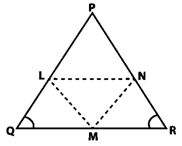Join L to M, M to N, N to L
PL = LQ, QM = MR and RN = NP (As L, M and N are mid-points of PQ, QR and RP)
PQ = QR
PL = LQ = QM = MR = PN = LR ________(i) (by mid-point theorem)
MN ∥ PQ
MN = PQ/2
MN = PL = LQ _______(ii)
LN ∥ QR
LN = QR/2
LN = QM = MR _______(iii)
From equation (i), (ii) and (iii), we get
PL = LQ = QM = MR = MN = LN
LN = MN
Hence Proved

Exercise 10.5

Question 1: ABC is a triangle and D is the mid-point of BC. The perpendiculars from D to AB and AC are equal. Prove that the triangle is isosceles.
Solution:
It is given that D is the midpoint of BC.
PD = DQ in a triangle ABC.In △BDP and △CDQ
PD = QD (Given)
BD = DC (D is mid-point)
∠BPD = ∠CQD (each 90°)
△BDP ≅ △CDQ (By RHS Criterion)
BP = CQ (CPCT) ________(i)
In △APD and △AQD
PD = QD (given)
APD = AQD (each 90°)
△APD ≅ △AQD (By RHS Criterion)
PA = QA (CPCT) ________(ii)
BP + PA = CQ + QA
AB = AC
Hence it is proved that two sides of the triangle are equal, so ABC is an isosceles.

Question 2: ABC is a triangle in which BE and CF are, respectively, the perpendiculars to the sides AC and AB. If BE = CF, prove that Δ ABC is isosceles
Solution:
ABC is a triangle in which BE and CF are perpendicular to the sides AC and AB respectively s.t. BE = CF.In Δ BCF and Δ CBE,
∠BFC = ∠CEB (each 90°)
BC = CB [Common side]
And CF = BE (Given)
ΔBFC ≅ ΔCEB (By RHS congruence criterion)
∠ FBC = ∠ EBC (CPCT)
∠ABC = ∠ACB (CPCT)
AC = AB (Opposite sides to equal angles are equal in a triangle)
Two sides of triangle ABC are equal.
Hence, ΔABC is isosceles.

Question 3: If perpendiculars from any point within an angle on its arms are congruent. Prove that it lies on the bisector of that angle.
Solution:It is given that, an angle ABC and BP is the mid-point of angle B.
Construction: Draw perpendicular PN and PM on the sides BC and BA.
In Δ BPM and Δ BPN
∠ BMP = ∠ BNP (each 90°)
BP = BP (Common)
MP = NP (given)
ΔBPM ≅ ΔBPN (By RHS congruence criterion)
∠MBP = ∠NBP (CPCT)
BP is the angular bisector of ∠ABC.
Hence proved

Question 4:  In figure, AD ⊥ CD and CB ⊥ CD. If AQ = BP and DP = CQ, prove that ∠DAQ = ∠CBP.
AD ⊥ CD and CB ⊥ CD.
Solution: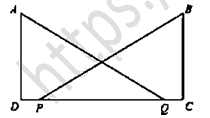It is given that, in the figure, AD ⊥ CD and CB ⊥ CD.
AQ = BP
DP = CQ ______(i)
PQ added on both sides of equation (i)
DP + PQ = PQ + QC
DQ = PC ... (i)
In ∆DAQ and ∆CBP
AQ = BP (given)
DQ = PC (proved above)
ΔDAQ ≅ ΔCBP ((by RHS congruence criterion)
∠DAQ = ∠CBP (CPCT)
Hence proved

Question 5: ABCD is a square, X and Y are points on sides AD and BC respectively such that AY = BX. Prove that BY = AX and ∠BAY = ∠ABX.
Solution: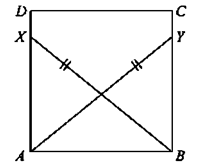It is given that ABCD is a square, X and Y are points on sides AD and BC respectively and AY = BX.
Join B and X, A and Y.
ABCD is a square,
∠DAB = ∠CBA (each 90°)
∠XAB = ∠YAB (each 90°)
In ∆XAB and ∆YBA
∠XAB = ∠YBA (each 90°)
BX = AY (given)
And AB = BA (Common)
ΔXAB ≅ ΔYBA (by RHS congruence criterion)
BY = AX (CPCT)
∠BAY = ∠ABX (CPCT)
Hence proved

Question 6:  Which of the following statements are true (T) and which are false (F):
(i) Sides opposite to equal angles of a triangle may be unequal.
(ii) Angles opposite to equal sides of a triangle are equal
(iii) The measure of each angle of an equilateral triangle is 60
(iv) If the altitude from one vertex of a triangle bisects the opposite side, then the triangle may be isosceles.
(v) The bisectors of two equal angles of a triangle are equal.
(vi) If the bisector of the vertical angle of a triangle bisects the base, then the triangle may be isosceles.
(vii) The two altitudes corresponding to two equal sides of a triangle need not be equal.
(viii) If any two sides of a right triangle are respectively equal to two sides of other right triangle, then the two triangles are congruent.
(ix) Two right-angled triangles are congruent if hypotenuse and a side of one triangle are respectively equal to the hypotenuse and a side of the other triangle.
Solution:
(i) False
(ii) True
(iii) True
(iv) False
(v) True
(vi) False
(vii) False
(viii) False
(ix) True

Question 7:  Fill the blanks.
In the following so that each of the following statements is true.
(i) Sides opposite to equal angles of a triangle are _________
(ii) Angle opposite to equal sides of a triangle are _________
(iii) In an equilateral triangle all angles are _________
(iv) In ΔABC, if ∠A = ∠C, then AB = _________
(v) If altitudes CE and BF of a triangle ABC are equal, then AB  _________
(vi) In an isosceles triangle ABC with AB = AC, if BD and CE are its altitudes, then BD is _________ CE.
(vii) In right triangles ABC and DEF, if hypotenuse AB = EF and side AC = DE, then ΔABC ≅ Δ _________
Solution:
(i) Sides opposite to equal angles of a triangle are equal.
(ii) Angles opposite to equal sides of a triangle are equal.
(iii) In an equilateral triangle all angles are equal.
(iv) In a ΔABC, if ∠A = ∠C, then AB = BC.
(v) If altitudes CE and BF of a triangle ABC are equal, then AB = AC
(vi) In an isosceles triangle ΔABC with AB = AC, if BD and CE are its altitudes, then BD is equal to CE
(vii) In right triangles ABC and DEF, if hypotenuse AB = EF and side AC = DE, then, ΔABC = ΔEFD.

Exercise 10.6
Question 1: In Δ ABC, if ∠A = 40° and ∠B = 60°. Determine the longest and shortest sides of the triangle.Solution:
It is given that, ΔABC, ∠A = 40° and ∠B = 60°
∠A + ∠B + ∠C = 180° (sum of angles in a triangle is 180°)
40° + 60° + ∠C = 180°
∠C = 180° – 100° = 80°
∠C = 80°
40° < 60° < 80°
∠A < ∠B < ∠C
∠C is greater angle and ∠ A is smaller angle.
∠A < ∠B < ∠C (side opposite to greater angle is larger and side opposite to smaller angle is smaller)
BC < AC < AB
Hence we can say that, AB is longest and BC is shortest side.

Question 2: In a Δ ABC, if ∠ B = ∠ C = 45°, which is the longest side?
Solution:
It is given that, ΔABC, ∠B = ∠C = 45°
∠A + ∠B + ∠C = 180° (Sum of angles in a triangle is 180°)
∠A + 90° = 180°
∠A = 180° – 90°
∠A = 90°
∠B = ∠C < ∠A
Hence, BC is the longest side.

Question 3: In Δ ABC, side AB is produced to D so that BD = BC. If ∠ B = 60° and ∠ A = 70°.
Solution:It is given that, Δ ABC, side AB is produced to D so that BD = BC ∠ B = 60°, and ∠ A = 70°
Construction: Join C and D
∠A + ∠B + ∠C = 180° (sum of angles in a triangle is 180°)
70° + 60° + ∠C = 180°
130° + ∠C = 180°
∠C = 180° – 130°
∠C = 50°
∠ACB = 50° ____________(i)
In ΔBDC
∠DBC = 180° – ∠ ABC
∠DBC = 180 – 60°
∠DBC = 120°
DBA is a straight line
BD = BC (given)
∠BCD = ∠BDC (angles opposite to equal sides are equal)
∠DBC + ∠BCD + ∠BDC = 180° (Sum of angles in a triangle is 180°)
120° + ∠ BCD + ∠ BCD = 180°
120° + 2∠BCD = 180°
2∠BCD = 180° – 120°
2∠BCD = 60°
∠BCD = 30°
∠BCD = ∠BDC = 30°  ____________(ii)
∠DAC = 70° (given)
∠ACD = ∠ ACB+ ∠ BCD
∠ACD = 50° + 30°
∠ACD = 80°
AC < DC < AD (Side opposite to greater angle is longer and smaller angle is smaller)
Hence proved

Question 4: Is it possible to draw a triangle with sides of length 2 cm, 3 cm and 7 cm?
Solution:
We know that, Sum of 2 sides > third side
Case 1
2 + 3 > 7
2 + 3 ≯ 7

Case 2
2 + 7 > 3
9 > 3

Case 3
3 + 7 > 2
10 > 2
All the 3 case should be true.
Hence, it is not possible to form a triangle.

Question 5:  O is any point in the interior of ΔABC. Prove that
(i) AB + AC > OB + OC
(ii) AB + BC + CA > OA + QB + OC
(iii) OA + OB + OC > (1/2) (AB + BC +CA)
Solution: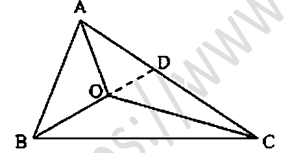(i) It is given that O is any point in the interior of ΔABC.
We know that, Sum of 2 sides > third side
In Δ ABC
AB + BC > AC
BC + AC > AB
AC + AB > BC
In ΔOBC
OB + OC > BC _______(i)
In ΔOAC
OA + OC > AC _______(ii)
In ΔOAB
OA + OB > AB _______(iii)
In ΔABD, we have
AB + AD > BO + OD _______(iv)  (BD = BO + OD)
In ΔODC, we have
OD + DC > OC _______ (v)

(i) By adding equation (iv) and (v)
AB + AD + OD + DC > BO + OD + OC
AB + (AD + DC) > OB + OC
AB + AC > OB + OC
we have
BC + BA > OA + OC ... (vii)
CA+ CB > OA + OB ... (viii)

(ii) Adding equation (vi), (vii) and (viii),
AB + AC + BC + BA + CA + CB > OB + OC + OA + OC + OA + OB
2AB + 2BC + 2CA > 2OA + 2OB + 2OC
2(AB + BC + CA) > 2(OA + OB + OC)
AB + BC + CA > OA + OB + OC

(iii) Adding equations (i), (ii) and (iii)
OB + OC + OA + OC + OA + OB > BC + AC + AB
2OA + 2OB + 2OC > AB + BC + CA
We get = 2(OA + OB + OC) > AB + BC +CA
(OA + OB + OC) > (1/2)(AB + BC +CA)

Question 6:  Prove that the perimeter of a triangle is greater than the sum of its altitudes.
Solution: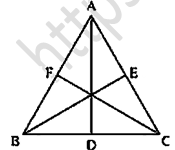It is given that, ΔABC in which AD ⊥ BC, BE ⊥ AC and CF ⊥ AB.
Therefore
AB + AC > 2AD _______(i) (perpendicular line segment is the shortest)
BE ⊥ AC
BA > BE and BC > BE
BA + BC > 2BE _______(ii) (perpendicular line segment is the shortest)
CF ⊥ AB
CA > CF and CB > CF
CA + CB > 2CF _______ (iii) (perpendicular line segment is the shortest)
By Adding equation (i), (ii) and (iii), we get
AB + AC + BA + BC + CA + CB > 2AD + 2BE + 2CF
2AB + 2BC + 2CA > 2(AD + BE + CF)
AB + BC + CA > AD + BE + CF
Hence we can say that the sum of its altitudes are smaller then the perimeter of the triangle.

Question 7:  In Fig., prove that:(i) CD + DA + AB + BC > 2AC
(ii) CD + DA + AB > BC
Solution:
To prove
(i) CD + DA + AB + BC > 2AC
(ii) CD + DA+ AB > BC
From the given figure,
We know that, in a triangle sum of any two sides is greater than the third side
(i) In ΔABC, we have
AB + BC > AC  ________(i)
CD + DA > AC ________ (ii)
By adding (i) and (ii), we get
AB + BC + CD + DA > AC + AC
AB + BC + CD + DA > 2AC

(ii) In Δ ABC, we have,
AB + AC > BC ________ (iii)
CD + DA > AC
CD + DA + AB > AC + AB
From equation (iii) and (iv), we get,
CD + DA + AB > AC + AB > BC
CD + DA + AB > BC
Hence proved

Question 8:  Which of the following statements are true (T) and which are false (F)?
(i) Sum of the three sides of a triangle is less than the sum of its three altitudes.
(ii) Sum of any two sides of a triangle is greater than twice the median drown to the third side
(iii) Sum of any two sides of a triangle is greater than the third side.
(iv) Difference of any two sides of a triangle is equal to the third side.
(v) If two angles of a triangle are unequal, then the greater angle has the larger side opposite to it
(vi) Of all the line segments that can be drawn from a point to a line not containing it, the perpendicular line segment is the shortest one.
Solution:
(i) False
(ii) True
(iii) True
(iv) False
(v) True
(vi) True

Question 9: Fill in the blanks to make the following statements true.
(i) In a right triangle the hypotenuse is the ___ side.
(ii) The sum of three altitudes of a triangle is ___ than its perimeter.
(iii) The sum of any two sides of a triangle is ___ than the third side.
(iv) If two angles of a triangle are unequal, then the smaller angle has the ___ side opposite to it.
(v) Difference of any two sides of a triangle is ___ than the third side.
(vi) If two sides of a triangle are unequal, then the larger side has ___ angle opposite to it.
Solution:
(i) In a right triangle the hypotenuse is the largest side
(ii) The sum of three altitudes of a triangle is less than its perimeter.
(iii) The sum of any two sides of a triangle is greater than the third side.
(iv) If two angles of a triangle are unequal, then the smaller angle has the smaller side opposite to it.
(v) Difference of any two sides of a triangle is less than the third side.
(vi) If two sides of a triangle are unequal, then the larger side has greater angle opposite to it.

Exercise VSAQs

Question 1: In two congruent triangles ABC and DEF, if AB = DE and BC = EF. Name the pairs of equal angles.
Solution:
It is given that, ∆ABC ≅ ∆DEF,
AB = DE and BC = EF
∠A = ∠D
∠B = ∠E
∠C = ∠F

Question 2: In two triangles ABC and DEF, it is given that ∠A = ∠D, ∠B = ∠ E and ∠ C = ∠F. Are the two triangles necessarily congruent?
Solution:
No.
Two triangles are not necessarily congruent, because we know only angle-angle-angle (AAA) criterion. This criterion can produce similar but not congruent triangles.

Question 3: If ABC and DEF are two triangles such that AC = 2.5 cm, BC = 5 cm, C = 75°, DE = 2.5 cm, DF = 5 cm and D = 75°. Are two triangles congruent?
Solution:
Yes
It is given that,
AC = DE (each 2.5cm)
BC = DF (each 5cm
∠D = ∠C (each 75°)
∆ABC ≅ ∆EDF (By SAS theorem triangle)

Question 4: In two triangles ABC and ADC, if AB = AD and BC = CD. Are they congruent?
Solution:
Yes
It is given that,
BC = CD (given)
AC = AC (common)
∆ABC ≅ ∆ADC (By SSS theorem triangle)

Question 5: In triangles ABC and CDE, if AC = CE, BC = CD, ∠A = 60°, ∠C = 30° and ∠D = 90°. Are two triangles congruent?
Solution:
Yes.
AC = CE (Given)
BC = CD (Given)
∠B = ∠D (each 90°)
∆ABC ≅ ∆CDE (By SSA criteria)

Question 6: ABC is an isosceles triangle in which AB = AC. BE and CF are its two medians. Show that BE = CF.
Solution:
It is given that AB = AC and BE and CF are two medians.
In △CFB and △BEC
CE = BF (Since, AC = AB =  (AC/2) = (AB/2) = CE = BF)
BC = BC (Common)
∠ECB = ∠FBC (Angle opposite to equal sides are equal)
△CFB ≅ △BEC (By SAS theorem)
BE = CF (CPCT)